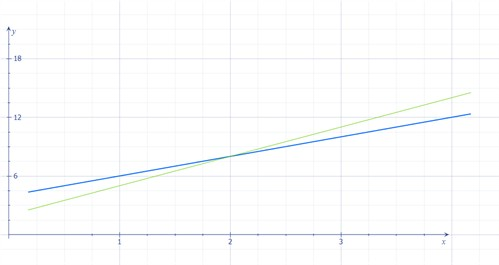# Solving systems of equations in two variables

A system of a linear equation comprises two or more equations and one seeks a common solution to the equations. In a system of linear equations, each equation corresponds with a straight line corresponds and one seeks out the point where the two lines intersect.

Example

Solve the following system of linear equations:

$\left\{\begin{matrix} y=2x+4\\ y=3x+2\\ \end{matrix}\right.$

Since we are seeking out the point of intersection, we may graph the equations:We see here that the lines intersect each other at the point x = 2, y = 8. This is our solution and we may refer to it as a graphic solution to the task.

But how does one reach a solution if the lines never intersect? One cannot, the system of equations have no solution.

One may also arrive at the correct answer with the help of the elimination method (also called the addition method or the linear combination method) or the substitution method.

When using the substitution method we use the fact that if two expressions y and x are of equal value x=y, then x may replace y or vice versa in another expression without changing the value of the expression.

Example

Solve the systems of equations using the substitution method

$\left\{\begin{matrix} y=2x+4\\ y=3x+2\\ \end{matrix}\right.$

We substitute the y in the top equation with the expression for the second equation:

$\begin{array}{lcl} 2x+4 & = & 3x+2\\ 4-2 & = & 3x-2x\\ 2 & = & x\\ \end{array}$

To determine the y-value, we may proceed by inserting our x-value in any of the equations. We select the first equation:

$y=2x+4$

We plug in x=2 and get

$y=2\cdot 2+4=8$

We have thus arrived at precisely the same answer as in the graphic solution.

The elimination method requires us to add or subtract the equations in order to eliminate either x or y, often one may not proceed with the addition directly without first multiplying either the first or second equation by some value.

Example

$2x-2y=8$

$x+y=1$

We now wish to add the two equations but it will not result in either x or y being eliminated. Therefore we must multiply the second equation by 2 on both sides and get:

$2x-2y=8$

$2x+2y=2$

Now we attempt to add our system of equations. We commence with the x-terms on the left, and the y-terms thereafter and finally with the numbers on the right side:

$(2x+2x)+(-2y+2y)=8+2$

The y-terms have now been eliminated and we now have an equation with only one variable:

$4x=10$

$x=\frac{10}{4}=2.5$

Thereafter, in order to determine the y-value we insert x=2.5 in one of the equations. We select the first:

$\begin{array}{lcl} 2\cdot 2.5-2y & = & 8\\ 5-8 & = & 2y\\ -3 & = & 2y\\ \frac{-3}{2} & = & y\\ y & = & -1.5\\ \end{array}$

## Video lesson

Solve the system of equations:

$\left\{\begin{matrix} 2x-4y=0\\ -4x+4y=-4 \end{matrix}\right.$